### The Potential Step

We wish to study the physics of a potential step for the case.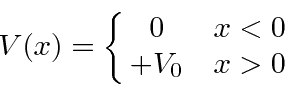For this problem, both regions have, so we will use the complex exponential solutions in both regions. This is essentially a 1D scattering problem. Assume there is a beam of particles with definite momentum coming in from the left and assume there is no flux of particles coming from the right.

For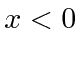, the solution isNote we have assumed the coefficient of the incident beam is 1. (Multiplying by some number does not change the physics.) Forthe solution is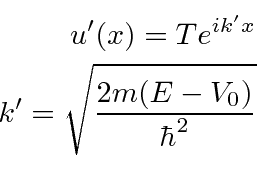(Note that a beam coming from the right, would have given aterm for.)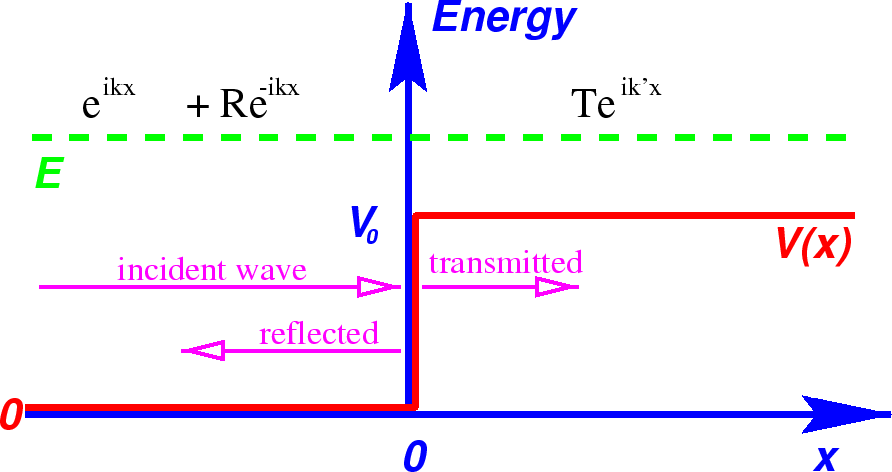There are two unknown coefficientsandwhich will be determined by matching boundary conditions. We will not require normalization to one particle, since we have a beam with definite momentum, which cannot be so normalized. (A more physical problem to solve would use an incoming wave packet with a spread in momentum.)

Continuity of the wave function atimpliesThe exponentials are all equal to 1 there so the equation is simple.

Continuity of the derivative of the wavefunction atgivesEvaluate and plug infrom the equation above. We can solve the problem.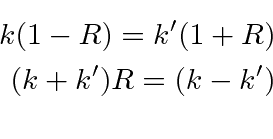The coefficients are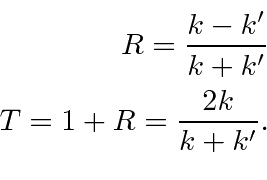We now have the full solution, given our assumption of particles incident from the left.Classically, all of the particles would be transmitted, continuing on to infinity.

In Quantum Mechanics, some probability is reflected.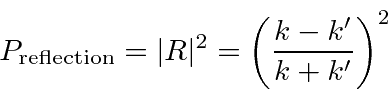(Note that we can simply use the coefficient ofbecause the incoming term has a coefficient of 1 and because the reflected particles are moving with the same velocity as the incoming beam.)

If we wish to compute the transmission probability, the easy way to do it is to say that itsWe'll get the same answers for the reflection and transmission coefficients using the probability flux to solve the problem.

The transmission probability goes to 1 one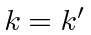(since there is no step). The transmission probability goes to 0 for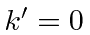(since the kinetic energy is zero).

Jim Branson 2013-04-22